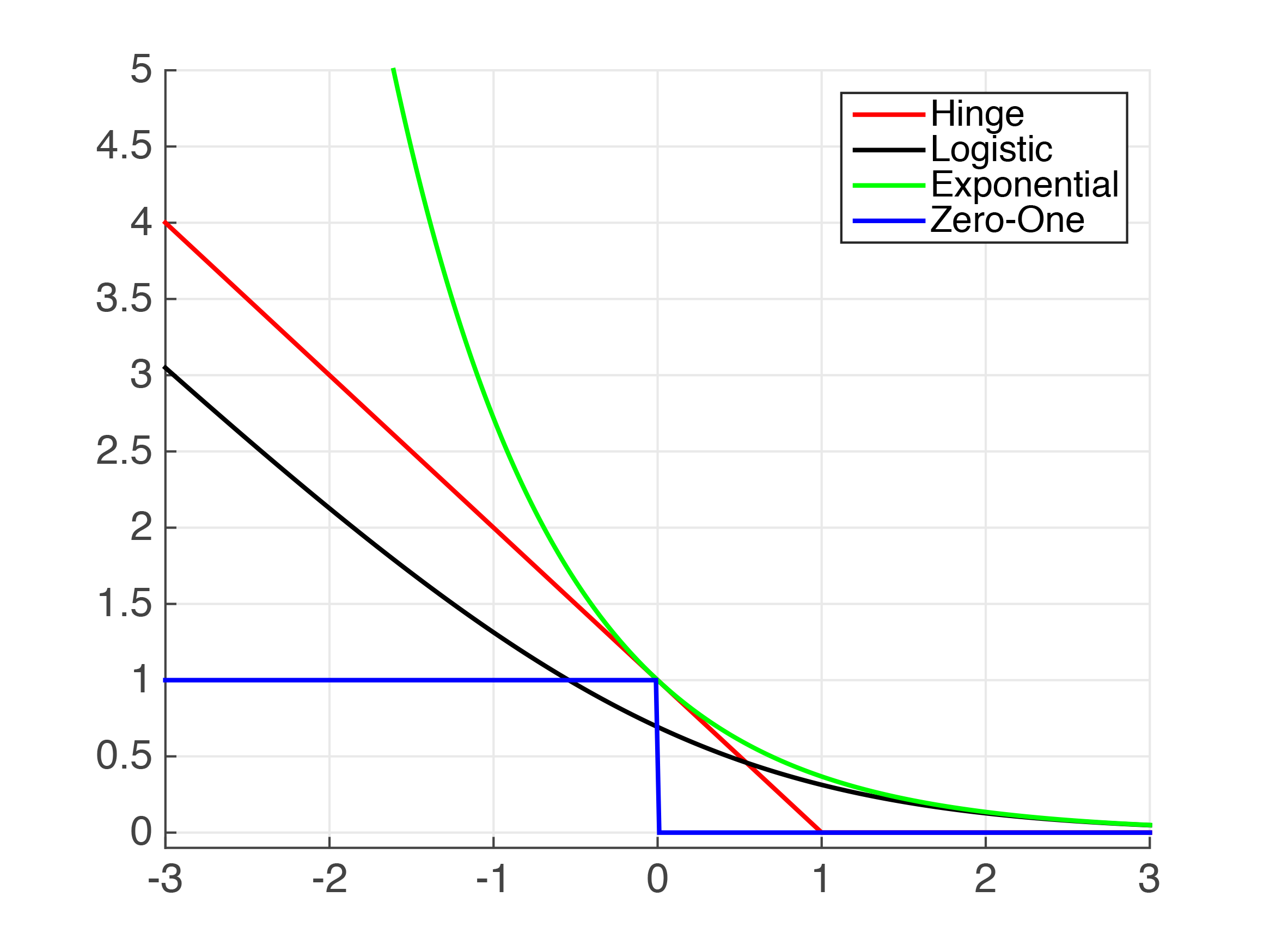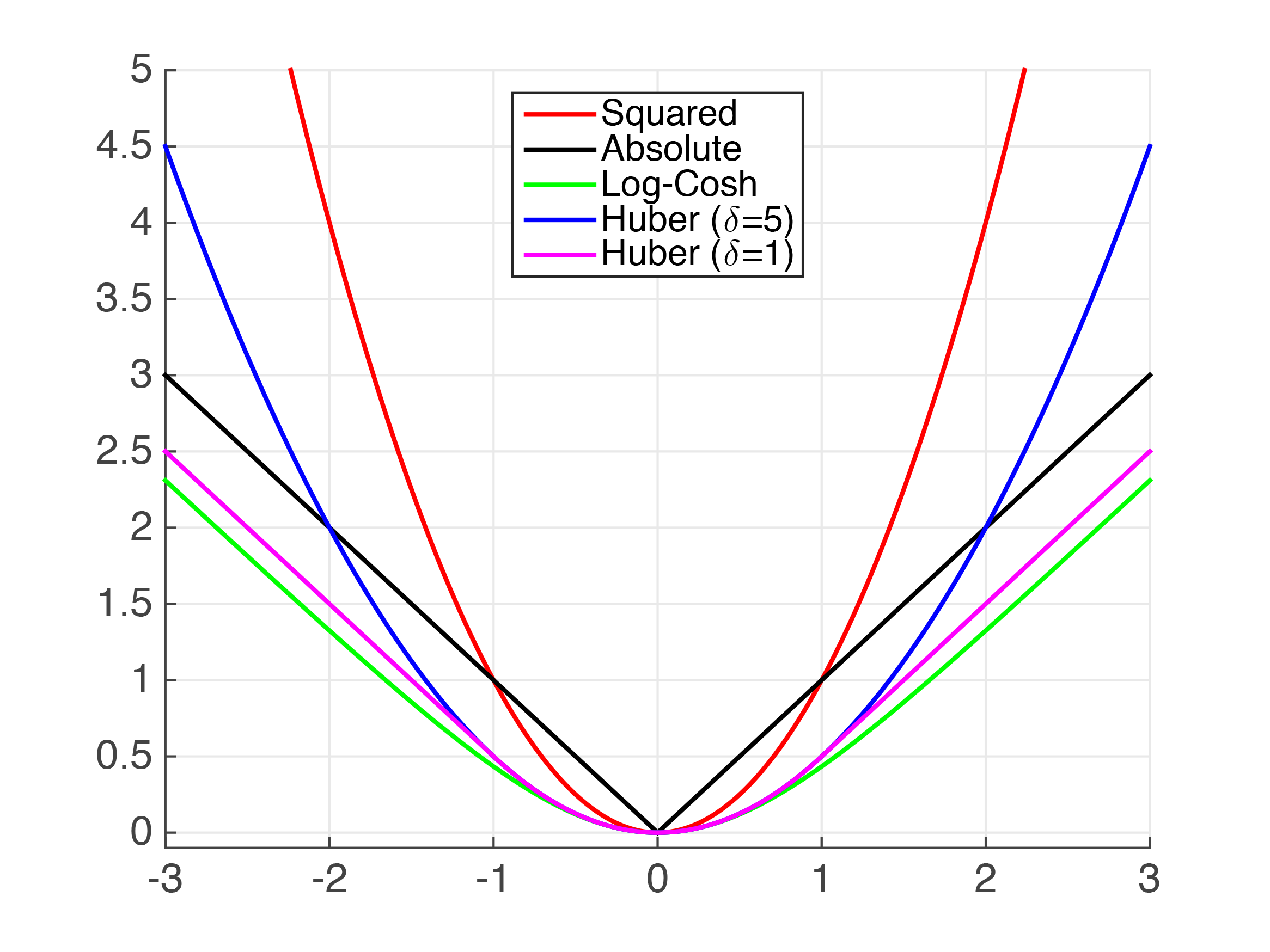## 10: Empirical Risk Minimization

Video II

### Recap

Remember the unconstrained SVM Formulation $\min_{\mathbf{w}}\ C\underset{Hinge-Loss}{\underbrace{\sum_{i=1}^{n}\max[1-y_{i}\underset{h({\mathbf{x}_i})}{\underbrace{(w^{\top}{\mathbf{x}_i}+b)}},0]}}+\underset{l_{2}-Regularizer}{\underbrace{\left\Vert w\right\Vert _{z}^{2}}}$ The hinge loss is the SVM's error function of choice, whereas the $\left.l_{2}\right.$-regularizer reflects the complexity of the solution, and penalizes complex solutions. This is an example of empirical risk minimization with a loss function $\ell$ and a regularizer $r$, $\min_{\mathbf{w}}\frac{1}{n}\sum_{i=1}^{n}\underset{Loss}{\underbrace{l(h_{\mathbf{w}}({\mathbf{x}_i}),y_{i})}}+\underset{Regularizer}{\underbrace{\lambda r(w)}},$ where the loss function is a continuous function which penalizes training error, and the regularizer is a continuous function which penalizes classifier complexity. Here, we define $\lambda$ as $\frac{1}{C}$ from the previous lecture.

### Commonly Used Binary Classification Loss Functions

Different Machine Learning algorithms use different loss functions; Table 4.1 shows just a few:
Loss $\ell(h_{\mathbf{w}}(\mathbf{x}_i,y_i))$UsageComments
Hinge-Loss
$\max\left[1-h_{\mathbf{w}}(\mathbf{x}_{i})y_{i},0\right]^{p}$
• Standard SVM($\left.p=1\right.$)
• (Differentiable) Squared Hingeless SVM ($\left.p=2\right.$)
• When used for Standard SVM, the loss function denotes the size of the margin between linear separator and its closest points in either class. Only differentiable everywhere with $\left.p=2\right.$.
Log-Loss $\left.\log(1+e^{-h_{\mathbf{w}}(\mathbf{x}_{i})y_{i}})\right.$Logistic Regression One of the most popular loss functions in Machine Learning, since its outputs are well-calibrated probabilities.
Exponential Loss $\left. e^{-h_{\mathbf{w}}(\mathbf{x}_{i})y_{i}}\right.$AdaBoost This function is very aggressive. The loss of a mis-prediction increases exponentially with the value of $-h_{\mathbf{w}}(\mathbf{x}_i)y_i$. This can lead to nice convergence results, for example in the case of Adaboost, but it can also cause problems with noisy data.
Zero-One Loss $\left.\delta(\textrm{sign}(h_{\mathbf{w}}(\mathbf{x}_{i}))\neq y_{i})\right.$ Actual Classification Loss Non-continuous and thus impractical to optimize.

Table 4.1: Loss Functions With Classification $\left.y\in\{-1,+1\}\right.$

Quiz: What do all these loss functions look like with respect to $\left.z=yh(\mathbf{x})\right.$?Figure 4.1: Plots of Common Classification Loss Functions - x-axis: $\left.h(\mathbf{x}_{i})y_{i}\right.$, or "correctness" of prediction; y-axis: loss value

Some questions about the loss functions:
1. Which functions are strict upper bounds on the 0/1-loss?
2. What can you say about the hinge-loss and the log-loss as $\left.z\rightarrow-\infty\right.$?
3. ### Commonly Used Regression Loss Functions

Regression algorithms (where a prediction can lie anywhere on the real-number line) also have their own host of loss functions:
Loss $\ell(h_{\mathbf{w}}(\mathbf{x}_i,y_i))$Comments
Squared Loss $\left.(h(\mathbf{x}_{i})-y_{i})^{2}\right.$
• Most popular regression loss function
• Estimates Mean Label
• DISADVANTAGE: Somewhat sensitive to outliers/noise
• Also known as Ordinary Least Squares (OLS)
Absolute Loss $\left.|h(\mathbf{x}_{i})-y_{i}|\right.$
• Also a very popular loss function
• Estimates Median Label
• ADVANTAGE: Less sensitive to noise
• DISADVANTAGE: Not differentiable at $0$
Huber Loss
• $\left.\frac{1}{2}\left(h(\mathbf{x}_{i})-y_{i}\right)^{2}\right.$ if $|h(\mathbf{x}_{i})-y_{i}|<\delta$,
• otherwise $\left.\delta(|h(\mathbf{x}_{i})-y_{i}|-\frac{\delta}{2})\right.$

• Also known as Smooth Absolute Loss
• ADVANTAGE: "Best of Both Worlds" of Squared and Absolute Loss
• Once-differentiable
• Takes on behavior of Squared-Loss when loss is small, and Absolute Loss when loss is large.
Log-Cosh Loss $\left.log(cosh(h(\mathbf{x}_{i})-y_{i}))\right.$, $\left.cosh(x)=\frac{e^{x}+e^{-x}}{2}\right.$
ADVANTAGE: Similar to Huber Loss, but twice differentiable everywhere

Table 4.2: Loss Functions With Regression, i.e. $\left.y\in\mathbb{R}\right.$

Quiz: What do the loss functions in Table 4.2 look like with respect to $\left.z=h(\mathbf{x}_{i})-y_{i}\right.$?Figure 4.2: Plots of Common Regression Loss Functions - x-axis: $\left.h(\mathbf{x}_{i})y_{i}\right.$, or "error" of prediction; y-axis: loss value

### Regularizers

When we look at regularizers it helps to change the formulation of the optimization problem to obtain a better geometric intuition: \begin{equation} \min_{\mathbf{w},b} \sum_{i=1}^n\ell(h_\mathbf{w}(\mathbf{x}),y_i)+\lambda r(\mathbf{w}) \Leftrightarrow \min_{\mathbf{w},b} \sum_{i=1}^n\ell(h_\mathbf{w}(\mathbf{x}),y_i) \textrm { subject to: } r(\mathbf{w})\leq B \end{equation} For each $\left.\lambda\geq0\right.$, there exists $\left.B\geq0\right.$ such that the two formulations in (4.1) are equivalent, and vice versa. In previous sections, $\left.l_{2}\right.$-regularizer has been introduced as the component in SVM that reflects the complexity of solutions. Besides the $\left.l_{2}\right.$-regularizer, other types of useful regularizers and their properties are listed in Table 4.3.
Regularizer $r(\mathbf{w})$Properties
$l_{2}$-Regularization
$\left.r(\mathbf{w}) = \mathbf{w}^{\top}\mathbf{w} = \|{\mathbf{w}}\|_{2}^{2}\right.$

• DISADVANTAGE: Uses weights on all features, i.e. relies on all features to some degree (ideally we would like to avoid this) - these are known as Dense Solutions.
$l_{1}$-Regularization $\left.r(\mathbf{w}) = \|\mathbf{w}\|_{1}\right.$
• Convex (but not strictly)
• DISADVANTAGE: Not differentiable at $0$ (the point which minimization is intended to bring us to
• Effect: Sparse (i.e. not Dense) Solutions
$l_p$-Norm $\left.\|{\mathbf{w}}\|_{p} = (\sum\limits_{i=1}^d v_{i}^{p})^{1/p}\right.$
• (often $\left.0<p\leq1\right.$)
• Initialization dependent

Table 4.3: Types of RegularizersFigure 4.3: Plots of Common Regularizers

### Famous Special Cases

This section includes several special cases that deal with risk minimization, such as Ordinary Least Squares, Ridge Regression, Lasso, and Logistic Regression. Table 4.4 provides information on their loss functions, regularizers, as well as solutions.
Ordinary Least Squares $\min_{\mathbf{w}} \frac{1}{n}\sum\limits_{i=1}^n (\mathbf{w}^{\top}x_{i}-y_{i})^{2}$
• Squared Loss
• No Regularization
• Closed form solution:
• $\left.\mathbf{w}=(\mathbf{X}\mathbf{X}^\top)^{-1}\mathbf{X}\mathbf{y}^{\top}\right.$
• $\left.\mathbf{X}=[\mathbf{x}_{1}, ..., \mathbf{x}_{n}]\right.$
• $\left.\mathbf{y}=[y_{1},...,y_{n}]\right.$
Ridge Regression $\min_{\mathbf{w}} \frac{1}{n}\sum\limits_{i=1}^n (\mathbf{w}^{\top}x_{i}-y_{i})^{2}+\lambda\|{w}\|_{2}^{2}$
• Squared Loss
• $l_{2}$-Regularization
• $\left.\mathbf{w}=(\mathbf{X}\mathbf{X}^{\top}+\lambda\mathbb{I})^{-1}\mathbf{X}\mathbf{y}^{\top}\right.$
Lasso $\min_{\mathbf{w}} \frac{1}{n}\sum\limits_{i=1}^n (\mathbf{w}^{\top}\mathbf{x}_{i}-{y}_{i})^{2}+\lambda\|\mathbf{w}\|_{1}$
• + sparsity inducing (good for feature selection)
• + Convex
• - Not strictly convex (no unique solution)
• - Not differentiable (at 0)
• Solve with (sub)-gradient descent or SVEN
Elastic Net $\min_{\mathbf{w}} \frac{1}{n}\sum\limits_{i=1}^n (\mathbf{w}^{\top}\mathbf{x}_{i}-{y}_{i})^{2}+\left.\alpha\|\mathbf{w}\|_{1}+(1-\alpha)\|{\mathbf{w}}\|_{2}^{2}\right.$ $\left.\alpha\in[0, 1)\right.$
• ADVANTAGE: Strictly convex (i.e. unique solution)
• + sparsity inducing (good for feature selection)
• + Dual of squared-loss SVM, see SVEN
Logistic Regression $\min_{\mathbf{w},b} \frac{1}{n}\sum\limits_{i=1}^n \log{(1+e^{-y_i(\mathbf{w}^{\top}\mathbf{x}_{i}+b)})}$
• Often $l_{1}$ or $l_{2}$ Regularized
• $\left.\Pr{(y|x)}=\frac{1}{1+e^{-y(\mathbf{w}^{\top}x+b)}}\right.$
Linear Support Vector Machine $\min_{\mathbf{w},b} C\sum\limits_{i=1}^n \max[1-y_{i}(\mathbf{w}^\top{\mathbf{x}_i+b}), 0]+\|\mathbf{w}\|_2^2$
• Typically $l_2$ regularized (sometimes $l_1$).
4.  In Bayesian Machine Learning, it is common to optimize $\lambda$, but for the purposes of this class, it is assumed to be fixed.